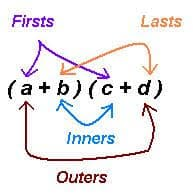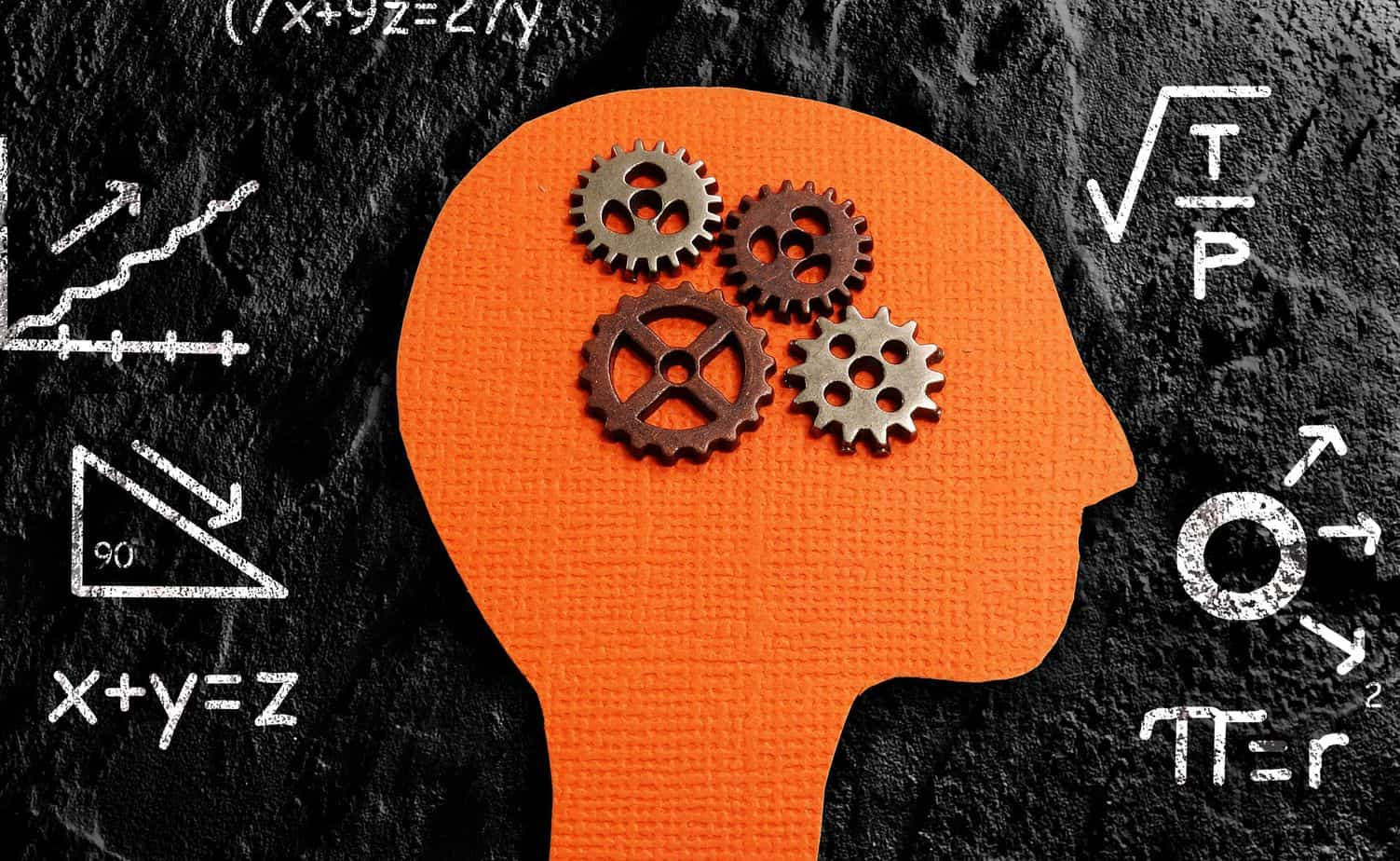# New Math/Old Math: Which is better?

WHICH IS BETTER?  THE NEW MATH OR THE OLD MATH?

The Old Math, or the older method of teaching math is criticized as it is focused on memorization, rather than understanding.  The New Math is described as a teaching method that promotes deeper understanding of math concepts.

For example, when multiplying two binomials, say, (a + b)(c + d), the Old Math teaches the FOIL method (First, Outside, Inside, Last).

It is the sum of:

• multiplying the First terms,
• multiplying the Outer terms,
• multiplying the Inner terms, and
• multiplying the Last terms

Example: (a+b)(c+d) = ac + ad + bc + bdThe New Math says “don’t teach FOIL” because it is just memorization of a method, or algorithm that applies to the multiplication of two binomials.  Rather, teach The Distributive Property of Multiplication which not only describes why FOIL works, but can be applied to many other types of multiplication problems.

(The Distributive Property states that when a number is multiplied by the sum of two numbers, the first number can be distributed to both of those numbers and multiplied by each of them separately, then adding the two products together for the same result as multiplying the first number by the sum.

Example: 2 X (3 + 5) = 2 X 3 + 2 X 5 = 16 and 2 X 8 = 16).So,

OLD MATH = MEMORIZATION OF ALGORITHMS

NEW MATH = DEEPER UNDERSTANDING OF MATH CONCEPTS

I was fortunate to have been taught a mix of The Old Math and The New Math when I was a student in public school.  So, I understand why we can have a mix of both.DO YOU WANT TO WRITE THE ALGORITHM OR WORK FOR AN ALGORITHM?

Let’s look at algorithms.  What is an algorithm?

a process or set of rules to be followed in calculations or other problem-solving operations, especially by a computer.”  (Dictionary.com)

Algorithms are becoming more and more a part of our daily lives.  Why? Because algorithms are used to “tell” computers what to do. Here is a good explanation of what algorithms are used for:

“To make a computer do anything, you have to write a computer program. To write a computer program, you have to tell the computer, step by step, exactly what you want it to do. The computer then ‘executes’ the program, following each step mechanically, to accomplish the end goal. When you are telling the computer what to do, you also get to choose how it’s going to do it. That’s where computer algorithms come in. The algorithm is the basic technique used to get the job done.”Some people argue that algorithms are taking over the world, or that they “work for an algorithm”.  Work for an algorithm? What does that mean? Well, Uber drivers don’t have supervisors, but they do have algorithms.  Uber increases its efficiency by managing over three million workers with an app that instructs drivers which passengers to pick up and which route to take.

So, does it follow that it you learn critical thinking (NEW MATH), you can write the algorithm, but if you memorize (OLD MATH), that means you’ll end up working for an algorithm?  I don’t think it’s quite so cut and dried.

In my current tutoring, I see students struggle because their adding and multiplication skills are poor.  Have we pushed the NEW MATH too far, so that we can’t quickly add numbers in our head? Is it WRONG to memorize 7X6 = 54?

I believe that critical thinking and deeper understanding will allow students to perform at higher levels, and ultimately, get the better jobs.  I also believe that as teachers and tutors, we can also go too far in supporting the NEW MATH. There has to be some allowance for learning short cuts, memorizing multiplication tables and simple algorithms like how to do long division.

Let’s have a mix of both:  old math and new math.Share with: